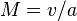# Mach number facts for kids

Kids Encyclopedia Facts

A mach number is a number that describes speed. It is a measure of the speed of an object compared to the speed of sound. Mach number is given as a percentage compared to the local speed of sound. For example, half the speed of sound = Mach 0.5.$M=v/a$

Where v is the speed of the object, and a is the speed of sound.

It is named after the Austrian physicist Ernst Mach. When writing a speed as a Mach number, the number comes after "Mach."Mach number Facts for Kids. Kiddle Encyclopedia.